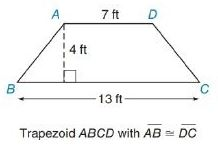Chapter 8.2, Problem 13EElementary Geometry For College St...

7th Edition
Alexander + 2 others
ISBN: 9781337614085

Solutions

Chapter
SectionElementary Geometry For College St...

7th Edition
Alexander + 2 others
ISBN: 9781337614085
Textbook Problem

In Exercises 13 to 18, find the area of the given polygon.To determine

To Find:

The area of the trapezium.

Explanation

Formula Used:

The area A of a trapezoid whose bases have lengths b1 and b2 and whose altitude has length h is given by A=12h(b1+b2)

Calculation:

It is given that the trapezium ABCD with AB-DC-. Therefore the given trapezium is an isosceles trapezium.

The two parallel bases of the trapezoids are AD=7 ft and BC=13 ft with the altitude AE and DF as 4 ft.

Still sussing out bartleby?

Check out a sample textbook solution.

See a sample solution

The Solution to Your Study Problems

Bartleby provides explanations to thousands of textbook problems written by our experts, many with advanced degrees!

Get Started

Place the following set of n = 20 scores in a frequency distribution table.

Essentials of Statistics for The Behavioral Sciences (MindTap Course List)

Find for .

Study Guide for Stewart's Multivariable Calculus, 8th

Which is the best graph of r = 1 − sin θ for 0 ≤ θ ≤ π?

Study Guide for Stewart's Single Variable Calculus: Early Transcendentals, 8th